##### The Japanese Bonsai specialist
Direct order Contact Help / Services Newsletter# Ophiopogon planiscapus 'nigrescens' 0.8 litre pot

Ophiopogon planiscapus nigrescens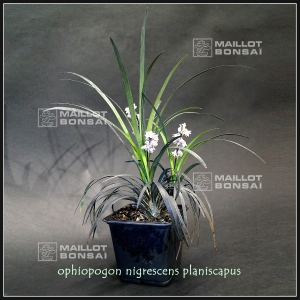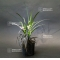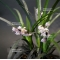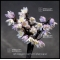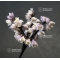ref. : 4720

5,00

Available quantity : 10Order

###### Description

Vivacious plant. Leaves 10/15-cm long and 0.4 cm wide. Strong tuft delivered in 0.8 liter plastic pot.pot.

Ophiopogon planiscapus ‘Nigrescens’ is the “Black mondo grass”, an herbaceous perennial in the Asparagaceae family, and its grass-like blades are very black when it grows in full-sun. Small white-to-pink flowers bloom on leafless stems that rise above the foliage. Even if there are few, they are followed by glossy pea-sized purple berries. The name ophiopogon comes from Greek ophis meaning “serpent” and “pogon” meaning “beard”. Planiscarpus refers to the flattened scape or flowers stalk;

for example a tulip blooms a top a scape (from Latin scapus for “shaft”). Mondo grass is also commonly known as lilyturf, but the “mondo” name is an older genus name of unexplained origin, certainly not a japanese one. Ophiopogon’s name in Japan is , meaning “black dragon”.koku ryu

#ophiopogon 6 #name 4.4 #planiscapus 4.1 #meaning 3.7 #small 3.5 #grass 3.5 #nigrescens 3 #kusamono 2.8 #flowers 2.7 #plants 2.6

Formule
(( ROUND((CHAR_LENGTH(b.article_nom)-CHAR_LENGTH(REPLACE(b.article_nom, 'ophiopogon', '')))/LENGTH('ophiopogon')) + ROUND((CHAR_LENGTH(b.article_description)-CHAR_LENGTH(REPLACE(b.article_description, 'ophiopogon', '')))/LENGTH('ophiopogon')) ) * 6) + (( ROUND((CHAR_LENGTH(b.article_nom)-CHAR_LENGTH(REPLACE(b.article_nom, 'name', '')))/LENGTH('name')) + ROUND((CHAR_LENGTH(b.article_description)-CHAR_LENGTH(REPLACE(b.article_description, 'name', '')))/LENGTH('name')) ) * 4.4) + (( ROUND((CHAR_LENGTH(b.article_nom)-CHAR_LENGTH(REPLACE(b.article_nom, 'planiscapus', '')))/LENGTH('planiscapus')) + ROUND((CHAR_LENGTH(b.article_description)-CHAR_LENGTH(REPLACE(b.article_description, 'planiscapus', '')))/LENGTH('planiscapus')) ) * 4.1) + (( ROUND((CHAR_LENGTH(b.article_nom)-CHAR_LENGTH(REPLACE(b.article_nom, 'meaning', '')))/LENGTH('meaning')) + ROUND((CHAR_LENGTH(b.article_description)-CHAR_LENGTH(REPLACE(b.article_description, 'meaning', '')))/LENGTH('meaning')) ) * 3.7) + (( ROUND((CHAR_LENGTH(b.article_nom)-CHAR_LENGTH(REPLACE(b.article_nom, 'grass', '')))/LENGTH('grass')) + ROUND((CHAR_LENGTH(b.article_description)-CHAR_LENGTH(REPLACE(b.article_description, 'grass', '')))/LENGTH('grass')) ) * 3.5) + (( ROUND((CHAR_LENGTH(b.article_nom)-CHAR_LENGTH(REPLACE(b.article_nom, 'nigrescens', '')))/LENGTH('nigrescens')) + ROUND((CHAR_LENGTH(b.article_description)-CHAR_LENGTH(REPLACE(b.article_description, 'nigrescens', '')))/LENGTH('nigrescens')) ) * 3) + (( ROUND((CHAR_LENGTH(b.article_nom)-CHAR_LENGTH(REPLACE(b.article_nom, 'flowers', '')))/LENGTH('flowers')) + ROUND((CHAR_LENGTH(b.article_description)-CHAR_LENGTH(REPLACE(b.article_description, 'flowers', '')))/LENGTH('flowers')) ) * 2.7) + (( ROUND((CHAR_LENGTH(b.article_nom)-CHAR_LENGTH(REPLACE(b.article_nom, 'mondo', '')))/LENGTH('mondo')) + ROUND((CHAR_LENGTH(b.article_description)-CHAR_LENGTH(REPLACE(b.article_description, 'mondo', '')))/LENGTH('mondo')) ) * 2.5) + (( ROUND((CHAR_LENGTH(b.article_nom)-CHAR_LENGTH(REPLACE(b.article_nom, 'scape', '')))/LENGTH('scape')) + ROUND((CHAR_LENGTH(b.article_description)-CHAR_LENGTH(REPLACE(b.article_description, 'scape', '')))/LENGTH('scape')) ) * 2.5) + (( ROUND((CHAR_LENGTH(b.article_nom)-CHAR_LENGTH(REPLACE(b.article_nom, 'litre', '')))/LENGTH('litre')) + ROUND((CHAR_LENGTH(b.article_description)-CHAR_LENGTH(REPLACE(b.article_description, 'litre', '')))/LENGTH('litre')) ) * 2.5)

## Secure payment## Delivery

Our logistic partners :